Latest Banking jobs   »   Quantitative Aptitude Quiz For SBI Clerk...

# Quantitative Aptitude Quiz For SBI Clerk Prelims 2021- 5th May

Directions (1-5): In each question two equations numbered (I) and (II) are given. You have to solve both the equations and mark appropriate answer.
(a) If x<y
(b) If x>y
(c) If x≥y
(d) If x≤y
(e) If x=y or no relation can be established.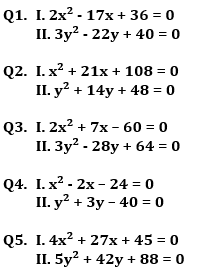(a) x > y
(b) x ≥ y
(c) x < y
(d) x ≤ y
(e) x = y or no relation can be established between x and y.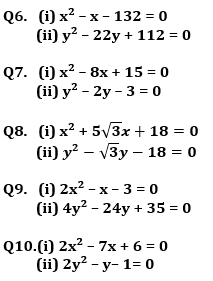Directions (11-15): In each question two equations numbered (I) and (II) are given. You should solve both the equations and mark appropriate answer.
(a) If x=y or no relation can be established
(b) If x>y
(c) If x<y
(d) If x≥y
(e) If x≤y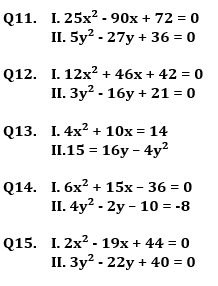Practice More Questions of Quantitative Aptitude for Competitive Exams:

###### SBI Clerk Study Plan 2021

Solutions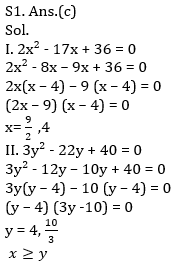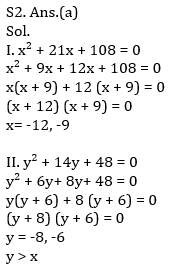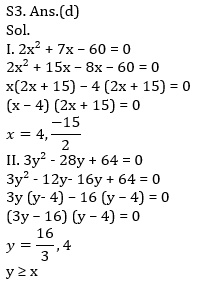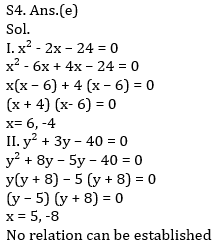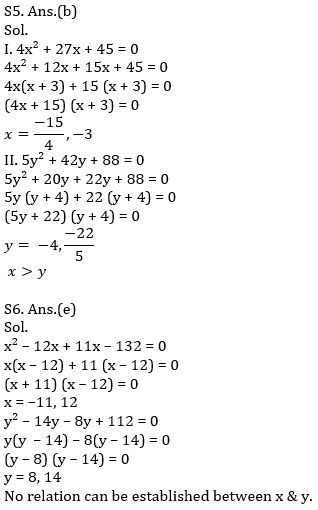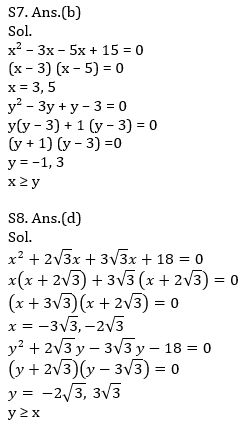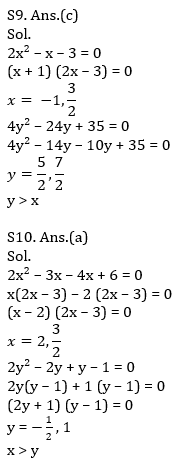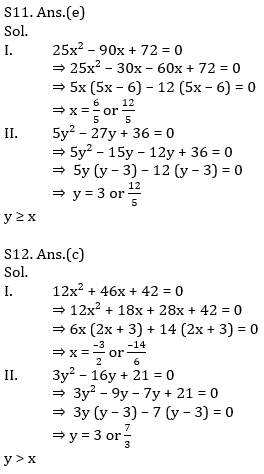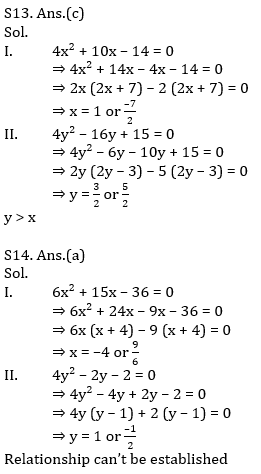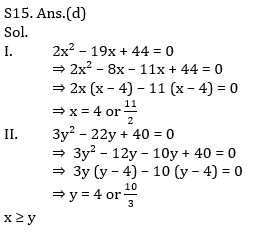#### Congratulations!